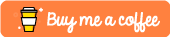#Getting Started With Tries in Python

This tutorial uses Python 3.6 in order to convey the concepts that I will be covering.

A `Trie` in computer science is a tree structure that allows you to do things such as very quick lookup of words within the english language. Typically if you were to write a word processor that did spell checks against words in a document, you would implement a `trie` and perform a very quick lookup to check whether or not the words in your word document are indeed valid words.

# Implementing a Very Simple Trie

When it comes to implementing a `trie` we typically use a series of nested hash tables. In Python the closest thing we have to a hash table is a `dict()` which enables us to store over 1 billion different key-value pairs, enough for the 172,000 currently employed in the English language.

Let’s take a quick look at how we would implement a simple `trie` structure in Python. We’ll define a `make_trie()` function which will take in a list of words and then from this list of words, it will create a `trie` structure.

``````_end = '*'

# takes in a list of words
def make_trie(*words):
# creates our root dict()
trie = dict()

for word in words:
# create a temporary dict based off our root
# dict object
temp_dict = trie

for letter in word:
# update our temporary dict and add our current
# letter and a sub-dictionary
temp_dict = temp_dict.setdefault(letter, {})

# If our word is finished, add {'*': '*'}
# this tells us our word is finished
temp_dict[_end] = _end
return trie

# Test our trie creation
trie = make_trie('hi', 'hello', 'howdy')
# print out our new trie
print(trie)``````

If we then attempt to run this you will see that we create a `trie` that has one root element: `h`.

`````` \$ python3.6 trie.py
{'h': {'i': {'*': '*'}, 'e': {'l': {'l': {'o': {'*': '*'}}}}, 'o': {'w': {'d': {'y': {'*': '*'}}}}}}``````

# Implementing a find_word() function

So now that we have created a `trie` structure we need to implement a mechanism that checks to see if a word exists within our `trie`.

``````def find_word(trie, word):
sub_trie = trie
for letter in word:
if letter in sub_trie:
sub_trie = sub_trie[letter]
else:
return False
else:
if _end in sub_trie:
return True
else:
return False``````

If we wanted to implement a function that took in an existing `trie` and returned a new `trie` that contained a new word that has been passed in, we could do something similar to this:

``````def add_word(trie, word):
# We want to catch if a word already
# exists within our trie structure
if find_word(trie, word):
# if it does just return our original trie
return trie

# if it doesn't we want to add the new word
temp_trie = trie
for letter in word:
# iterate through our word and see how
# much of the word we already have within our
# structure
if letter in temp_trie:
temp_trie = temp_trie[letter]
else:
temp_trie = temp_trie.setdefault(letter, {})

# Terminate our new word
temp_trie[_end] = _end
# return our new trie object
return temp_trie``````

# Complete Code Sample

Below you’ll find the complete code sample for this tutorial. I’ve fleshed this out into a Python class that you can use much like you would a `list` or a `queue`.

``````class Trie():

def __init__(self):
self._end = '*'
self.trie = dict()

def __repr__(self):
return repr(self.trie)

def make_trie(self, *words):
trie = dict()
for word in words:
temp_dict = trie
for letter in word:
temp_dict = temp_dict.setdefault(letter, {})
temp_dict[self._end] = self._end
return trie

def find_word(self, word):
sub_trie = self.trie

for letter in word:
if letter in sub_trie:
sub_trie = sub_trie[letter]
else:
return False
else:
if self._end in sub_trie:
return True
else:
return False

if self.find_word(word):
return self.trie

temp_trie = self.trie
for letter in word:
if letter in temp_trie:
temp_trie = temp_trie[letter]
else:
temp_trie = temp_trie.setdefault(letter, {})
temp_trie[self._end] = self._end
return temp_trie

my_trie = Trie()
print(my_trie)

print(my_trie.find_word("hi"))
print(my_trie.find_word("how"))